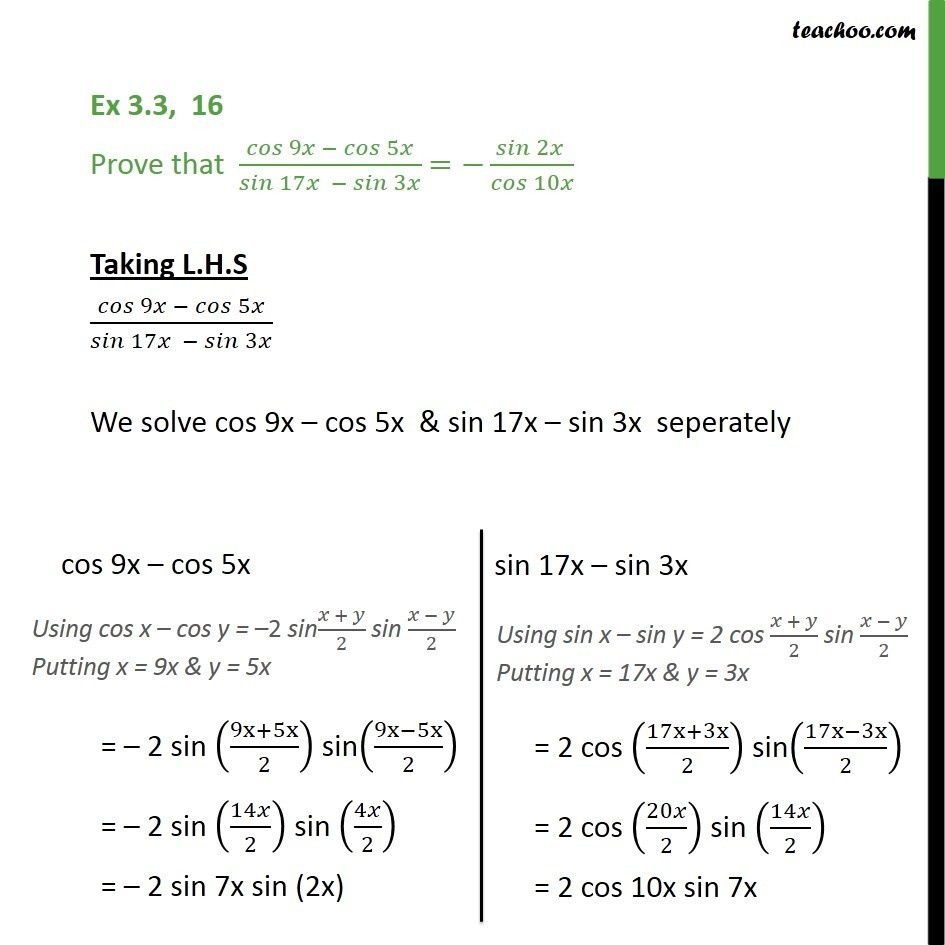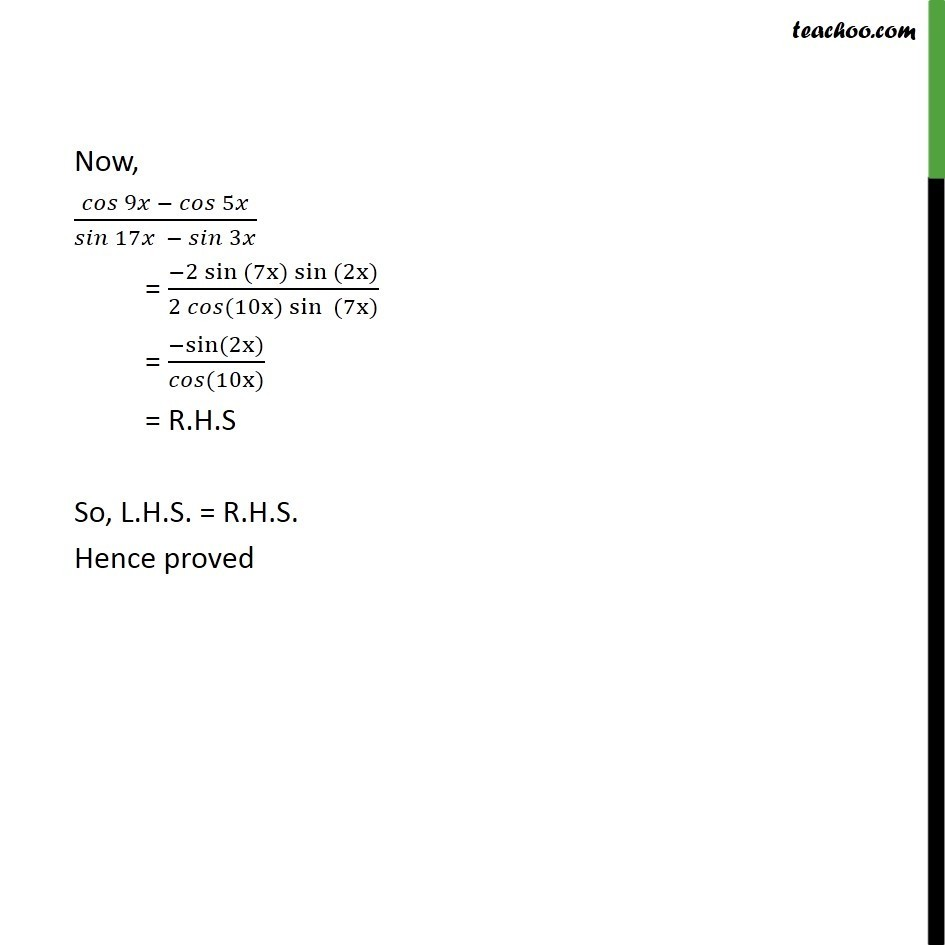Subscribe to our Youtube Channel - https://you.tube/teachoo

1. Chapter 3 Class 11 Trigonometric Functions
2. Concept wise
3. Cos x + cos y formula

Transcript

Ex 3.3, 16 Prove that 9 5 /( 17 3 ) = 2 / 10 Taking L.H.S 9 5 /( 17 3 ) We solve cos 9x cos 5x & sin 17x sin 3x seperately Now, 9 5 /( 17 3 ) = ( 2 sin (7x) sin (2x) )/(2 (10x)sin (7x) ) = sin (2x) / (10x) = R.H.S So, L.H.S. = R.H.S. Hence proved

Cos x + cos y formula